# Important Definitions & Formulas: Polynomials Notes | Study Mathematics (Maths) Class 10 - Class 10

## Class 10: Important Definitions & Formulas: Polynomials Notes | Study Mathematics (Maths) Class 10 - Class 10

The document Important Definitions & Formulas: Polynomials Notes | Study Mathematics (Maths) Class 10 - Class 10 is a part of the Class 10 Course Mathematics (Maths) Class 10.
All you need of Class 10 at this link: Class 10

Polynomial

An algebraic expression of the form p(x) = a0 + a1 x + a2 x2 + a3 x3 + ...... + an xn, in which the variables involved have only non-negative integral exponents, is called a polynomial in x of degree n.

Note:

In the polynomial a0 + a1 x + a2 x2 + .... + an xn

1. an ≠ 0

2. a0, a1 x1, a2 x2, a3 x3, ..... an - 1 xn - 1, anxn are terms.

3. a0, a1, a2, ..... an - 1, an are the co-efficients of x0, x1, x2, ....., xn-1, xn respectively.

Degree of a Polynomial

The highest power of the variable in a polynomial is called its degree

Example: 5x + 3 is a polynomial in x of degree 1.

p(y) = 3y2 + 4y - 4 is a polynomial in y of degree 2.

• Linear Polynomial: A polynomial of degree 1 is called a linear polynomial. A linear polynomial is generally written as ax + b (a ≠ 0), where a, b are real coefficients.
• Quadratic Polynomial: A polynomial of degree 2 is called a quadratic polynomial. A quadratic polynomial is generally written as ax2 + bx + c (a ≠ 0), where a, b and c are real coefficients.
• Cubic Polynomial: A polynomial of degree 3 is called a cubic polynomial. A cubic polynomial is generally written as ax3 + bx2 + cx + d (a ≠ 0), where a, b, c and d are real coefficients.

Examples: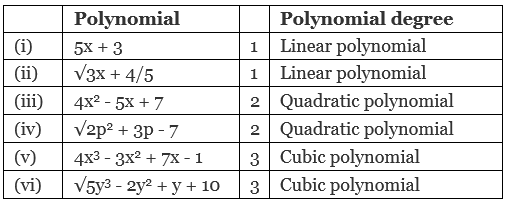Try yourself:The degree of the polynomial, x4 – x2 +2 is

Value of a Polynomial at a Given Point

If p (x) is a polynomial in x and ‘a’ is a real number. Then the value obtained by putting x = a in p (x) is called the value of p (x) at x = a.

Example: Let p(x) = 5x2 - 4x + 2 then its value at x = 2 is given by

p(2) = 5 (2)2 - 4 (2) + 2 = 5 (4) - 8 + 2 = 20 - 8 + 2 = 14

Thus, the value of p(x) at x = 2 is 14.

Zeroes of a Polynomial

A real number ‘a’ is said to be a zero of the polynomial p (x), if p (a) = 0.

Example: Let p (x) = x2 - x - 2 Then p (2) = (2)2 - (2) - 2 = 4 - 4 = 0,

and p (-1) = (-1)2 - (-1) - 2 = 2 - 2 = 0

∴ (-1) and (2) are the zeroes of the polynomial x- x - 2.

Note:

I. A linear polynomial has at the most one zero.
II. A quadratic polynomial has at the most two zeroes.

III. In general a polynomial of degree n has at the most n zeroes.

Geometrical Meaning of the Zeroes of a Polynomial

First, we consider a linear polynomial p (x) = ax + b.

Let ‘k’ be a zero, then p(k) = ak + b = 0

⇒ ak + b = 0

⇒ ak = - b or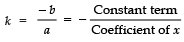The graph of a linear polynomial is always a straight line. It may or may not pass through the x-axis. In case the graph line is passing through a point on the x-axis, then the y-coordinate of that point must be zero. In general, for a linear polynomial ax + b = 0, (a ≠ 0), the graph is a straight line that can intersect the x-axis at exactly one point, namely,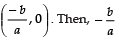is the zero of the polynomial ax + b.

In the given figure, CD is meeting x-axis at x = -1.

∴ Zero of ax + b is -1.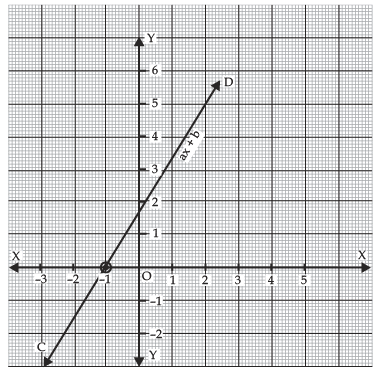Note:

A zero of a linear polynomial is the x-coordinate of the point, where the graph intersects the x-axis.

The graph of ax2 + bx + c, (a ≠0) is a curve of ∪ shape, called a parabola.

• If a > 0 in ax2 + bx + c, the shape of the parabola is ∪ (opening upwards).
• If a < 0 in ax2 + bx + c, the shape of parabola is ∩ (opening downwards).

In the given figure, the graph of a quadratic polynomial x2 - 3x - 4 is shown. It intersects x-axis at (-1, 0) and (4, 0). Therefore, its zeroes are -1 and 4. Here, a > 0, so the graph opens upwards.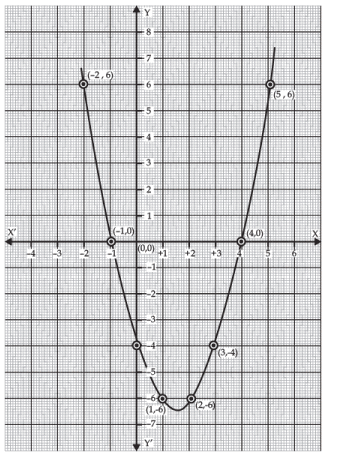Whereas the following figure is a graph of the polynomial - x2 + x + 6. Since it intersects the x-axis at (3, 0) and (-2, 0). Therefore, the zeroes of - x2 + x + 6 are -2 and 3.

Here a < 0, so the parabola opens downwards.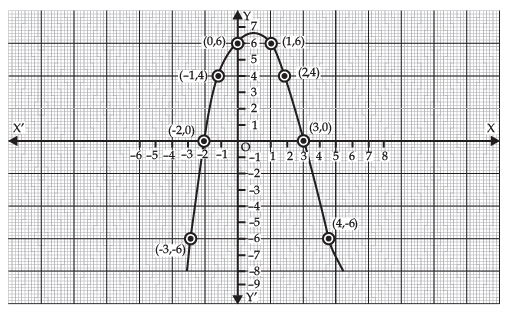Note: In the case of Quadratic polynomial - at most 2 zeroes, Cubic polynomial - at most 3 zeroes, Biquadratic polynomial - at most 4 zeroes.

Relationship between Zeroes and Coefficients of Polynomials

p(x) = ax2 + bx + c, α + β = -b/a, αβ = c/a,
where α and β are the zeroes of the polynomial p(x) = ax2 + bx + c
• For a cubic polynomial,
p(x) = ax3 + bx2 + cx + d,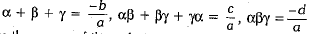where α, β and γ are the zeroes of the polynomial p(x) = ax3 + bx2 + cx + d.
Try yourself:What is the quadratic polynomial whose sum and the product of zeroes is √2, ⅓ respectively?

Division Algorithm
If we divide a polynomial p(x) by a polynomial g(x), then their exists polynomials q(x) and r(x) such that, p(x) = g(x) x q(x) + r(x), where r(x) = 0 or deg r(x) < deg g(x).

To divide one polynomial by another, follow the steps given below.

Step 1: Arrange the terms of the dividend and the divisor in the decreasing order of their degrees.

Step 2: To obtain the first term of the quotient, divide the highest degree term of the dividend by the highest degree term of the divisor  Then carry out the division process.

Step 3: The remainder from the previous division becomes the dividend for the next step. Repeat this process until the degree of the remainder is less than the degree of the divisor.

The document Important Definitions & Formulas: Polynomials Notes | Study Mathematics (Maths) Class 10 - Class 10 is a part of the Class 10 Course Mathematics (Maths) Class 10.
All you need of Class 10 at this link: Class 10Use Code STAYHOME200 and get INR 200 additional OFF

## Mathematics (Maths) Class 10

58 videos|354 docs|138 tests

Track your progress, build streaks, highlight & save important lessons and more!

,

,

,

,

,

,

,

,

,

,

,

,

,

,

,

,

,

,

,

,

,

;